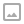## Python - Generator

·   ·  ☕ 4 min read

### Python - Generator

Python provides a generator to create your own iterator function. A generator is a special type of function which does not return a single value, instead it returns an iterator object with a sequence of values. In a generator function, a yield statement is used rather than a return statement. The following is a simple generator function.

def myGenerator():
print('First item')
yield 10

print('Second item')
yield 20

print('Last item')
yield 30


In the above example, myGenerator() is a generator function. It uses yield instead of return keyword. So, this will return the value against the yield keyword each time it is called. However, you need to create an iterator for this function, as shown below.

>>> gen = myGenerator()
>>> next(gen)
First item
10
>>> next(gen)
Second item
20
>>> next(gen)
Last item
30


The generator function cannot include the return keyword. If you include it then it will terminate the function. The difference between yield and return is that yield returns a value and pauses the execution while maintaining the internal states, whereas the return statement returns a value and terminates the execution of the function.

The following generator function includes the return keyword.
Example: Generator Function

def myGenerator():
print('First item')
yield 10

return

print('Second item')
yield 20

print('Last item')
yield 30


Now, execute the above function as shown below.

>>> gen = myGenerator()
>>> next(gen)
First item
10
>>> next(gen)
Traceback (most recent call last):
File "<pyshell#13>", line 1, in <module>
it.__next__()
StopIteration


As you can see, the above generator stops executing after getting the first item because the return keyword is used after yielding the first item.

The generator function can also use the for loop.
Example: Generator Function with For Loop

def getSequenceUpTo(x):
for i in range(x):
yield i


As you can see above, the getSequenceUpTo function uses the yield keyword. The generator is called just like a normal function. However, its execution is paused on encountering the yield keyword. This sends the first value of the iterator stream to the calling environment. However, local variables and their states are saved internally.

The above generator function myGenerator can be called as below.

>>> seq = getSequenceUpTo(5)
>>> next(seq)
0
>>> next(seq)
1
>>> next(seq)
2
>>> next(seq)
3
>>> next(seq)
4
>>> next(seq)
Traceback (most recent call last):
File "<pyshell#13>", line 1, in <module>
it.__next__()
StopIteration


The function resumes when next() is issued to the iterator object. The function finally terminates when next() encounters the StopIteration error.

In the following example, function squareOfSequence() acts as a generator. It yields the square of a number successively on every call of next().
Example:

def squareOfSequence(x):
for i in range(x):
yield i*i


The following script shows how to call the above generator function.

gen=squareOfSequence(5)
while True:
try:
except StopIteration:
break


The above script uses the try..except block to handle the StopIteration error. It will break the while loop once it catches the StopIteration error.
Result:

Received on next(): 0


We can use the for loop to traverse the elements over the generator. In this case, the next() function is called implicitly and StopIteration is also automatically taken care of.
Example: Generator with the For Loop

squres = squareOfSequence(5)
for sqr in squres:
print(sqr)


Result:

0
1
4
9
16


Note:

One of the advantages of the generator over the iterator is that elements are generated dynamically. Since the next item is generated only after the first is consumed, it is more memory efficient than the iterator.

### Generator Expression

Python also provides a generator expression which is a shorter way of defining simple generator functions. Generator expression is an anonymous generator. The following is a generator expression for the squareOfSequence() function.

>>> squres = (x*x for x in range(5))
>>> print(next(squre))
0
>>> print(next(squre))
1
>>> print(next(squre))
4
>>> print(next(squre))
9
>>> print(next(squre))
16


In the above example, (x*x for x in range(5)) is a generator expression. The first part of an expression is the yield value and the second part is the for loop with the collection.

The generator expression can also be passed in a function. It should be passed without parentheses, as shown below.

>>> import math
>>> sum(x*x for x in range(5))
30


In the above example, a generator expression is passed without parentheses into the built-in function sum.WRITTEN BY
Ohidur Rahman Bappy
📚Learner 🐍 Developer